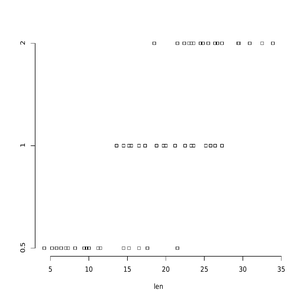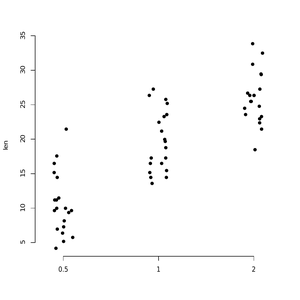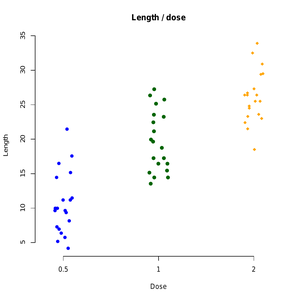# Create One Dimensional Scatterplots in R Programming – stripchart() Function

• Last Updated : 20 Jul, 2020

Strip Chart is defined as one dimensional scatter plots or dot plots which is used as an alternative to box plot when sample sizes are small. It is created using the `stripchart()` function in R Language.

Syntax:
stripchart(x, data = NULL method, jitter )

Parameters:
x: value of data from which the plots are to be produced.
data: a data.frame from which the variables in x value should be taken.
Method: the method is basically used to separate coincident points.
jitter: when method = “jitter” is used, jitter will produce the amount of jittering applied.

Approach:

• This example make use of ToothGrowth data base
• stripchart() Function is implemented using ToothGrowth data base.

Dataset:

 `ToothGrowth\$dose <- ``as.factor``(ToothGrowth\$dose)`` ` `# Print the first 7 rows``head``(ToothGrowth, 7)`

Output:

```   len supp dose
1  4.2   VC  0.5
2 11.5   VC  0.5
3  7.3   VC  0.5
4  5.8   VC  0.5
5  6.4   VC  0.5
6 10.0   VC  0.5
7 11.2   VC  0.5```

Above code will print data set

Example 1: Simple Stripchart

 `# Plot len by dose``stripchart``(len ~ dose, data = ToothGrowth,``         ``pch = 22, frame = ``FALSE``)`

Output:Example 2: Plot vertical stripchart

 `# Vertical plot using method = "jitter"``stripchart``(len ~ dose, data = ToothGrowth,``         ``pch = 16, frame = ``FALSE``, vertical = ``TRUE``,``         ``method = ``"jitter"``)`

Output:Example 3: Change in point shapes (pch) and colors by groups

 `# Change point shapes (pch) and colors by groups``# add main title and axis labels``stripchart``(len ~ dose, data = ToothGrowth,``         ``frame = ``FALSE``, vertical = ``TRUE``,``         ``method = ``"jitter"``, pch = ``c``(16, 19, 18),``         ``col = ``c``(``"blue "``, ``"darkgreen"``, ``"orange"``),``         ``main = ``"Length / dose"``, xlab = ``"Dose"``, ylab = ``"Length"``)`

Output:Above example will add the main title and axis labels and print colored stripcharts.

My Personal Notes arrow_drop_up